Tech

# Excel Cure for Hospitals Works for All Monte Carlo Modeling

A cardiac doctor’s question about Monte Carlo simulations and generating random columns using Excel applies to all industries and companies looking...

Dr. James B. sent in this week’s question. James works in a hospital cardiac unit and wants to do some Monte-Carlo simulations on hospital census statistics. He wants to generate two random columns, one with admission date and one with discharge date. He tried using RANDBETWEEN in both columns, but then sometimes the discharge date falls before the admission date.

Using RANDBETWEEN
The RANDBETWEEN() function will take two whole numbers and generate a random number between those numbers. Here are some examples of the function

=RANDBETWEEN(1,10) will generate a random number between 1 and 10 inclusive.

=RANDBETWEEN(-5,5) will generate a random number between -5 and 5.

The function always produces a whole number without decimal places. If you need to return some decimal places, you can ask for a number that is 100 times larger and then divide by 100, like this:

=RANDBETWEEN(80,120)/100 will generate a random percentage between 80% and 120%. Note that this will never produce 89.1% all of the results will be an exact percentage without decimals, something like 80%, 81%, 82%, and so on.

If you want to generate random percentages that might return 80.12%, use =RANDBETWEEN(8000,12000)/10000.

Using RANDBETWEEN for Dates
Using the function with dates is a little bit trickier. You cannot enter the date directly in the formula. For example, =RANDBETWEEN(1/1/2012,3/31/2012) will result in a #NUM! error.

Instead, specify the start and end date using the DATE function. To generate a random admission date between January 1 and March 31, 2012, you would use this formula:

=RANDBETWEEN(DATE(2012,1,1),DATE(2012,3,31)).

In some situations, there are shorter formulas. To generate a random date in January 2012, use:

=DATE(2012,1,RANDBETWEEN(1,31)).

This formula chooses a random number between 1 and 31 and uses it for the day argument in the DATE function.

(There is an arcane loophole. Because the DATE function can convert the 45th day of January 2012 to February 14, 2012, you could also use =DATE(2012,1,RANDBETWEEN(1,91) to generate random dates between January 1 and March 31, 2012.)

If you want to generate random dates that range from tomorrow until 14 days from tomorrow, you could use =TODAY()+RANDBETWEEN(1,15).

Generating the Census Data
Let’s say the doctor needs to generate 100 random admission dates during the month of December 2011. The formula for cells A2:A101 is =DATE(2011,11,30)+RANDBETWEEN(1,31), as shown in Figure 1.

Fig. 1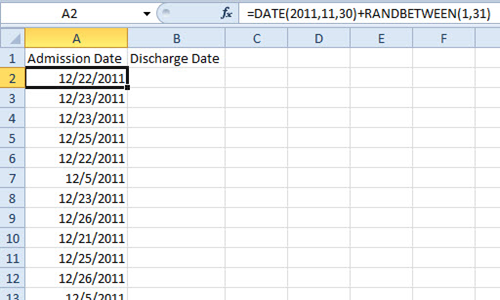If the length of patient stay runs from 2 days to 15 days, the formula in column B would be =A2+RANDBETWEEN(2,15), as in Figure 2.

Fig. 2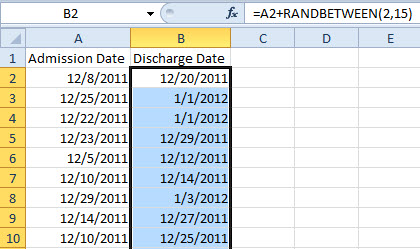If no discharges ever happen on a weekend, the formula in B could be: =WORKDAY(A2,RANDBETWEEN(2,10)). This will generate discharge dates on only Monday through Friday dates, as in Figure 3.

Fig. 3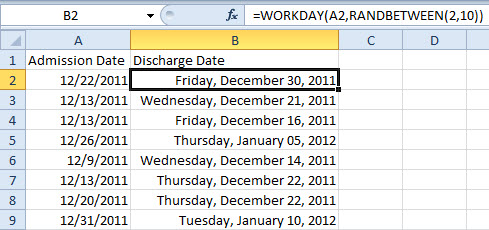To prevent discharges on holidays, use the optional third argument for the WORKDAY function as shown in Figure 4.

Fig. 4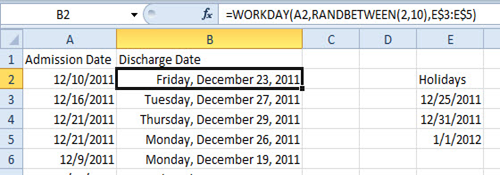The inherent problem with this model is that it assumes someone is just as likely to stay 15 days as he is to stay 2 days. In reality, James might notice that the length of stay tends to be grouped around a mean with a certain standard deviation. If most people end up staying in the cardiac unit 6, 7, or 8 days, you might find that average stay is 7 days with a standard deviation of two days.

Rather than using RANDBETWEEN(2,15), the model would improve if you used =NORM.INV. This function requires a percentage between 0% and 99.999%, as well as a mean and standard deviation. Rather than returning numbers that are a scattershot between 2 and 15, the NORM.INV will return random numbers that are grouped around the mean. Figure 5 shows the result of NORM.INV for various percentages.

Fig. 5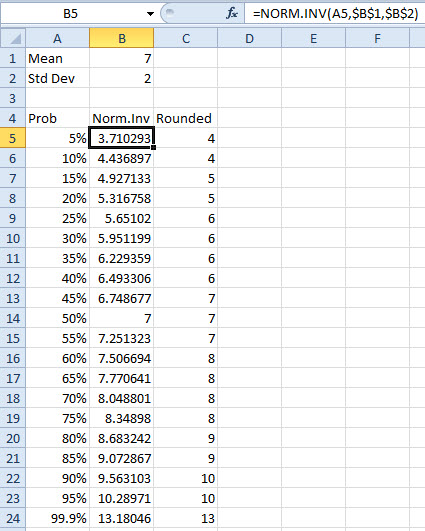To utilize NORM.INV in the hospital census model, you would change the formula in B to =A2+ROUND(NORM.INV(RAND(),7,2),0). In this case, the old RAND() function returns a random decimal between 0 and 1, as shown in Figure 6.

Fig. 6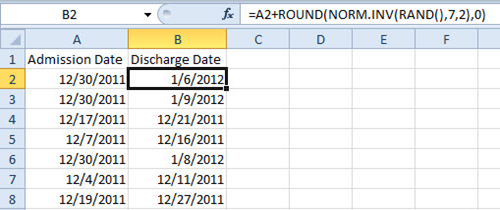Every time you press the F9 key, the model will generate new random numbers.

CFO contributor and Microsoft MVP Bill Jelen is the author of 34 books about Microsoft Excel. He and Wayne Eckerson will be teaming up on December 8th to deliver a webcast on dashboards, and how to design and then build these highly useful tools using Excel.